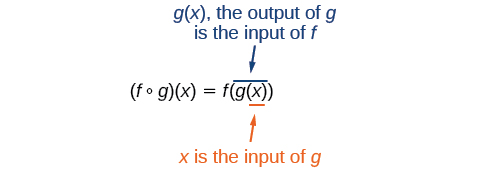3.4 Composition of functions  (Page 2/9)

 Page 2 / 9

Performing algebraic operations on functions

Find and simplify the functions $\text{\hspace{0.17em}}\left(g-f\right)\left(x\right)\text{\hspace{0.17em}}$ and $\text{\hspace{0.17em}}\left(\frac{g}{f}\right)\left(x\right),\text{\hspace{0.17em}}$ given $\text{\hspace{0.17em}}f\left(x\right)=x-1\text{\hspace{0.17em}}$ and $\text{\hspace{0.17em}}g\left(x\right)={x}^{2}-1.\text{\hspace{0.17em}}$ Are they the same function?

Begin by writing the general form, and then substitute the given functions.

No, the functions are not the same.

Note: For $\text{\hspace{0.17em}}\left(\frac{g}{f}\right)\left(x\right),\text{\hspace{0.17em}}$ the condition $\text{\hspace{0.17em}}x\ne 1\text{\hspace{0.17em}}$ is necessary because when $\text{\hspace{0.17em}}x=1,\text{\hspace{0.17em}}$ the denominator is equal to 0, which makes the function undefined.

Find and simplify the functions $\text{\hspace{0.17em}}\left(fg\right)\left(x\right)\text{\hspace{0.17em}}$ and $\text{\hspace{0.17em}}\left(f-g\right)\left(x\right).$

Are they the same function?

$\begin{array}{l}\left(fg\right)\left(x\right)=f\left(x\right)g\left(x\right)=\left(x-1\right)\left({x}^{2}-1\right)={x}^{3}-{x}^{2}-x+1\\ \left(f-g\right)\left(x\right)=f\left(x\right)-g\left(x\right)=\left(x-1\right)-\left({x}^{2}-1\right)=x-{x}^{2}\end{array}$

No, the functions are not the same.

Create a function by composition of functions

Performing algebraic operations on functions combines them into a new function, but we can also create functions by composing functions. When we wanted to compute a heating cost from a day of the year, we created a new function that takes a day as input and yields a cost as output. The process of combining functions so that the output of one function becomes the input of another is known as a composition of functions . The resulting function is known as a composite function . We represent this combination by the following notation:

$\left(f\circ g\right)\left(x\right)=f\left(g\left(x\right)\right)$

We read the left-hand side as $“f\text{\hspace{0.17em}}$ composed with $\text{\hspace{0.17em}}g\text{\hspace{0.17em}}$ at $\text{\hspace{0.17em}}x,”$ and the right-hand side as $“f\text{\hspace{0.17em}}$ of $\text{\hspace{0.17em}}g\text{\hspace{0.17em}}$ of $\text{\hspace{0.17em}}x.”$ The two sides of the equation have the same mathematical meaning and are equal. The open circle symbol $\text{\hspace{0.17em}}\circ \text{\hspace{0.17em}}$ is called the composition operator. We use this operator mainly when we wish to emphasize the relationship between the functions themselves without referring to any particular input value. Composition is a binary operation that takes two functions and forms a new function, much as addition or multiplication takes two numbers and gives a new number. However, it is important not to confuse function composition with multiplication because, as we learned above, in most cases $f\left(g\left(x\right)\right)\ne f\left(x\right)g\left(x\right).$

It is also important to understand the order of operations in evaluating a composite function. We follow the usual convention with parentheses by starting with the innermost parentheses first, and then working to the outside. In the equation above, the function $\text{\hspace{0.17em}}g\text{\hspace{0.17em}}$ takes the input $\text{\hspace{0.17em}}x\text{\hspace{0.17em}}$ first and yields an output $\text{\hspace{0.17em}}g\left(x\right).\text{\hspace{0.17em}}$ Then the function $\text{\hspace{0.17em}}f\text{\hspace{0.17em}}$ takes $\text{\hspace{0.17em}}g\left(x\right)\text{\hspace{0.17em}}$ as an input and yields an output $\text{\hspace{0.17em}}f\left(g\left(x\right)\right).$In general, $\text{\hspace{0.17em}}f\circ g\text{\hspace{0.17em}}$ and $\text{\hspace{0.17em}}g\circ f\text{\hspace{0.17em}}$ are different functions. In other words, in many cases $\text{\hspace{0.17em}}f\left(g\left(x\right)\right)\ne g\left(f\left(x\right)\right)\text{\hspace{0.17em}}$ for all $\text{\hspace{0.17em}}x.\text{\hspace{0.17em}}$ We will also see that sometimes two functions can be composed only in one specific order.

For example, if $\text{\hspace{0.17em}}f\left(x\right)={x}^{2}\text{\hspace{0.17em}}$ and $\text{\hspace{0.17em}}g\left(x\right)=x+2,$ then

$\begin{array}{ccc}\hfill f\left(g\left(x\right)\right)& =& f\left(x+2\right)\hfill \\ & =& {\left(x+2\right)}^{2}\hfill \\ & =& {x}^{2}+4x+4\hfill \end{array}$

but

$\begin{array}{ccc}\hfill g\left(f\left(x\right)\right)& =& g\left({x}^{2}\right)\hfill \\ & =& {x}^{2}+2\hfill \end{array}$

These expressions are not equal for all values of $\text{\hspace{0.17em}}x,\text{\hspace{0.17em}}$ so the two functions are not equal. It is irrelevant that the expressions happen to be equal for the single input value $\text{\hspace{0.17em}}x=-\frac{1}{2}.$

Note that the range of the inside function (the first function to be evaluated) needs to be within the domain of the outside function. Less formally, the composition has to make sense in terms of inputs and outputs.

The sequence is {1,-1,1-1.....} has
how can we solve this problem
Sin(A+B) = sinBcosA+cosBsinA
Prove it
Eseka
Eseka
hi
Joel
June needs 45 gallons of punch. 2 different coolers. Bigger cooler is 5 times as large as smaller cooler. How many gallons in each cooler?
7.5 and 37.5
Nando
find the sum of 28th term of the AP 3+10+17+---------
I think you should say "28 terms" instead of "28th term"
Vedant
the 28th term is 175
Nando
192
Kenneth
if sequence sn is a such that sn>0 for all n and lim sn=0than prove that lim (s1 s2............ sn) ke hole power n =n
write down the polynomial function with root 1/3,2,-3 with solution
if A and B are subspaces of V prove that (A+B)/B=A/(A-B)
write down the value of each of the following in surd form a)cos(-65°) b)sin(-180°)c)tan(225°)d)tan(135°)
Prove that (sinA/1-cosA - 1-cosA/sinA) (cosA/1-sinA - 1-sinA/cosA) = 4
what is the answer to dividing negative index
In a triangle ABC prove that. (b+c)cosA+(c+a)cosB+(a+b)cisC=a+b+c.
give me the waec 2019 questions
the polar co-ordinate of the point (-1, -1)ByByByByBy